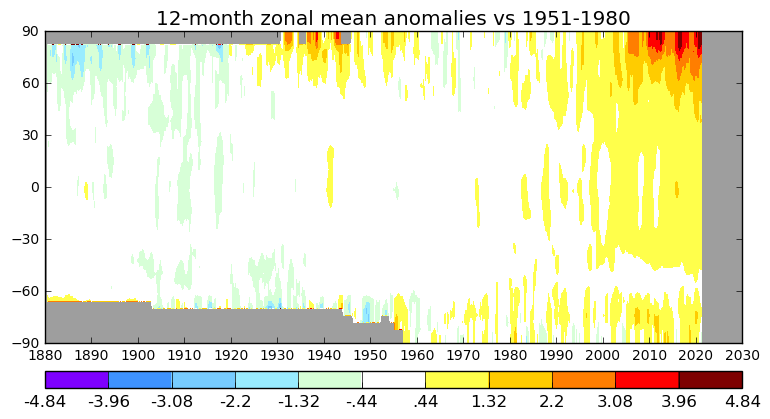# Just Warming Up

#### 2021-10-05"Just Warming Up", from The Empty Fields uses surface temperature data to render what climate change sounds like. The GISS (Godard Institute for Space Studies - not too far from Code Owl Studios, The Earthly Frames' HQ) Surface Temperature data set contains monthly mean measurements dating back to 1880. To transform this data to sound, first, a Python script mapped the temperatures to a range of MIDI notes, while also creating drones for years and decades averages.

`def step_average(sequence, step):`

`    """`

`    Creates a shorter, average list of numbers based on the desired steps`

`    Parameters`

`    ----------`

`        sequence: list`

`            List of integers or floats`

`        step: int`

`            The number of steps you wish to average. E.g. [1,2,3,4,5,6,7,8] with a step of 4 would return [10,24]`

`    Returns`

`    -------`

`        list`

`            The stepped list result`

`    """`

`    stepped_sequence = []`

`    step_sequence = []`

`    for sequence_number, sequence_item in enumerate(sequence, start=0):`

`        if sequence_number % step == 0 and sequence_number != 0:`

`            average = get_list_average(step_sequence)`

`            stepped_sequence.append(average)`

`            step_sequence = []`

`        step_sequence.append(sequence_item)`

`    return stepped_sequence`

This MIDI was fed through analog and virtual instruments to create the track. You can find the source code here.